Courses

# Test: Rational Numbers - 2

## 20 Questions MCQ Test Mathematics (Maths) Class 7 | Test: Rational Numbers - 2

Description
This mock test of Test: Rational Numbers - 2 for Class 7 helps you for every Class 7 entrance exam. This contains 20 Multiple Choice Questions for Class 7 Test: Rational Numbers - 2 (mcq) to study with solutions a complete question bank. The solved questions answers in this Test: Rational Numbers - 2 quiz give you a good mix of easy questions and tough questions. Class 7 students definitely take this Test: Rational Numbers - 2 exercise for a better result in the exam. You can find other Test: Rational Numbers - 2 extra questions, long questions & short questions for Class 7 on EduRev as well by searching above.
QUESTION: 1

### Which of the following is the product of (-7/8) and 4/21?

Solution:

Solution :- the product of -7/8 and 4/21 is

[(-7) * (8)] * [(4) * (21)]

= - 1/6

QUESTION: 2

Solution:
QUESTION: 3

### Rewrite −44/72 in the simplest form.

Solution:
QUESTION: 4

What should be added to -5/11 to get 3/11 ?

Solution:
QUESTION: 5

Which of the rational number is positive?

Solution:
QUESTION: 6

Write the rational number whose numerator is 4 × (– 7) and denominator is (3 –7) × (15 – 11).

Solution:
QUESTION: 7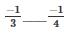Solution:

Since -¼ is  closer to zero than -⅓ , -¼ is greater than -⅓ . So the answer is A.

QUESTION: 8

0 ____ −7/6

Solution:
QUESTION: 9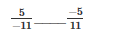Solution:
QUESTION: 10

What number should be added to 3/8 to get -1/24?

Solution:

3/8 + x = -1/24

x = -1/24 - 3/8

x = -10/24

x = -5/12

QUESTION: 11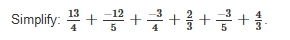Solution: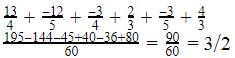QUESTION: 12

From his home, Rahul walks 6/7 km towards school and then returns 5/6 km on the same way towards his home to reach a landmark. Where will he be now from his home?

Solution:

We have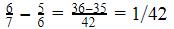km

QUESTION: 13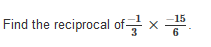Solution:
QUESTION: 14

Product of two rational numbers is−8/9, one is−10/3, find other

Solution:
QUESTION: 15

Reduce  − 63/99  to the standard form.

Solution:

Since its -63/99, dividing both numerator and denominator by 9 we get -63/9=-7 and 99/9=11 .
So it's -7/11

QUESTION: 16

Write the rational number whose denominator is the smallest 2 digit number and the numerator is the greatest 3 digit number.

Solution:
QUESTION: 17

The rational number 9/1 in integer is _____.

Solution:

If we have 1 in the denominator, the number is the number of numerator only. That is 9/1=9

QUESTION: 18

NUmertor of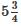is

Solution:
QUESTION: 19

Which of the following is the reciprocal of the reciprocal of a rational number?

Solution:
QUESTION: 20

Find out two rational numbers between −3/4 and 0.

Solution:

Since -2/4=-½ which is greater than -¾ and less than 0 and -¼ is also greater than -¾ and less than 0.